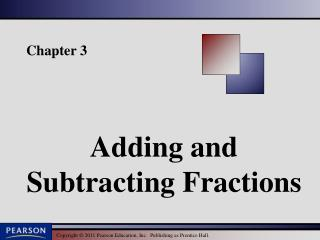DownloadDownload PresentationDownload Presentation- - - - - - - - - - - - - - - - - - - - - - - - - - - E N D - - - - - - - - - - - - - - - - - - - - - - - - - - -
##### Presentation Transcript

1. Chapter 3 Adding and Subtracting Fractions

2. Finding the Least Common Multiple Using Multiples Method 1: Find the LCM of a List of Numbers Using Multiples of the Largest Number Step 1: Write the multiples of the largest number (starting with the number itself) until a multiple common to all numbers in the list is found. Step 2: The multiple found in Step 1 is the LCM.

3. Example Find the LCM of 9 and 12. Write the multiples of 12 until we find a number that is also a multiple of 9. 12  1 = 12 Not a multiple of 9. 12  2 = 24 Not a multiple of 9. 12  3 = 36 A multiple of 9. The LCM of 9 and 12 is 36.

4. Finding the Least Common Multiple Using Multiples Method 2: Find the LCM of a List of Numbers Using Prime Factorization Step 1: Write the prime factorization of each number. Step 2: For each different prime factor in step 1, circle the greatest number of times that factor occurs in any one factorization. Step 3: The LCM is the product of the circle factors.

5. Example Find the LCM of 72 and 60. Circle the greatest number of prime factors found in either factorization. The LCM is the product of the circle factors.

6. Example Find the LCM of 15, 18, and 54.

7. Writing Equivalent Fractions To add or subtract unlike fractions, we first write equivalent fractions with the LCM as the denominator. To write an equivalent fraction, where a, b, and c are nonzero numbers.

8. Example Write an equivalent fraction with the indicated denominator.

9. Example Write an equivalent fraction with the indicated denominator.